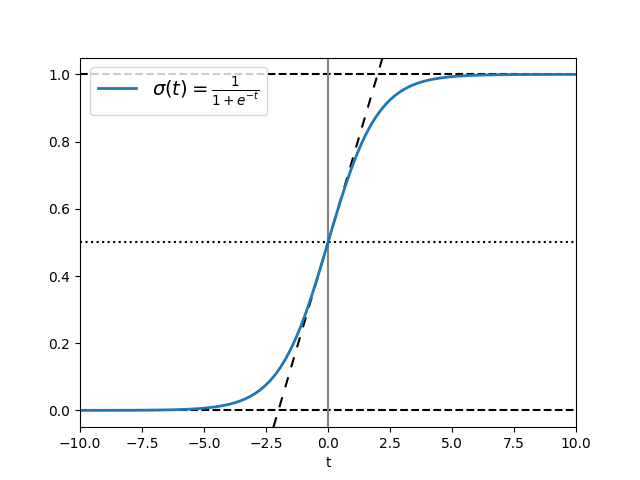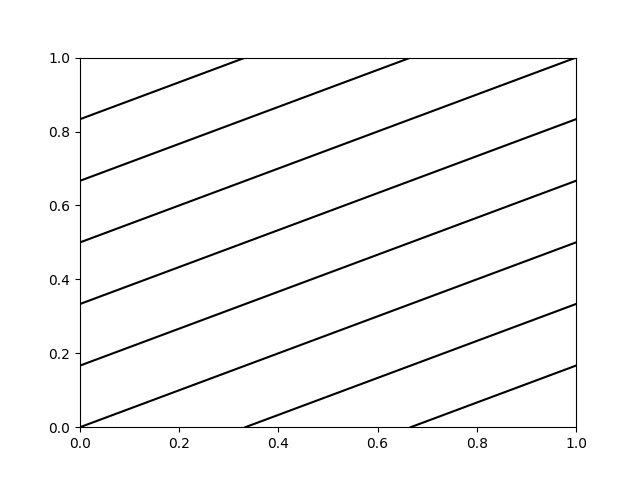# Infinite lines¶

axvline and axhline draw infinite vertical / horizontal lines, at given x / y positions. They are usually used to mark special data values, e.g. in this example the center and limit values of the sigmoid function.

axline draws infinite straight lines in arbitrary directions.

import numpy as np
import matplotlib.pyplot as plt

t = np.linspace(-10, 10, 100)
sig = 1 / (1 + np.exp(-t))

plt.axhline(y=0, color="black", linestyle="--")
plt.axhline(y=0.5, color="black", linestyle=":")
plt.axhline(y=1.0, color="black", linestyle="--")
plt.axvline(color="grey")
plt.axline((0, 0.5), slope=0.25, color="black", linestyle=(0, (5, 5)))
plt.plot(t, sig, linewidth=2, label=r"$\sigma(t) = \frac{1}{1 + e^{-t}}$")
plt.xlim(-10, 10)
plt.xlabel("t")
plt.legend(fontsize=14)
plt.show()axline can also be used with a transform parameter, which applies to the point, but not to the slope. This can be useful for drawing diagonal grid lines with a fixed slope, which stay in place when the plot limits are moved.

for pos in np.linspace(-2, 1, 10):
plt.axline((pos, 0), slope=0.5, color='k', transform=plt.gca().transAxes)

plt.ylim([0, 1])
plt.xlim([0, 1])
plt.show()References

The use of the following functions, methods, classes and modules is shown in this example:

Keywords: matplotlib code example, codex, python plot, pyplot Gallery generated by Sphinx-Gallery